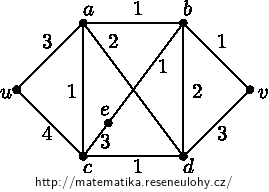## Dijkstra’s Algorithm

With the help of Dijkstra’s algorithm determine the shortest path form vertex $$u$$ to vertex $$v$$ in the following graphs.
Construct a table whose collumns will contain estimates of the distance from $$u$$ after each itteration of the algorithm.